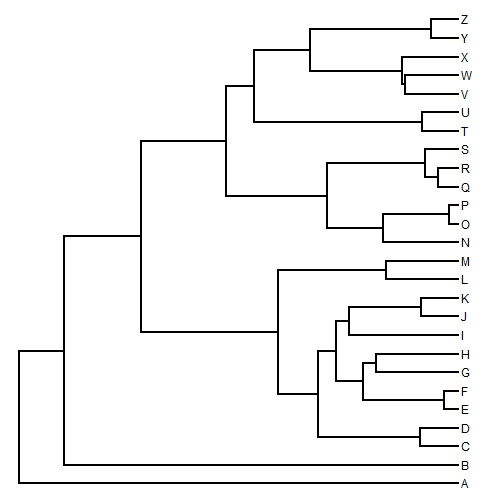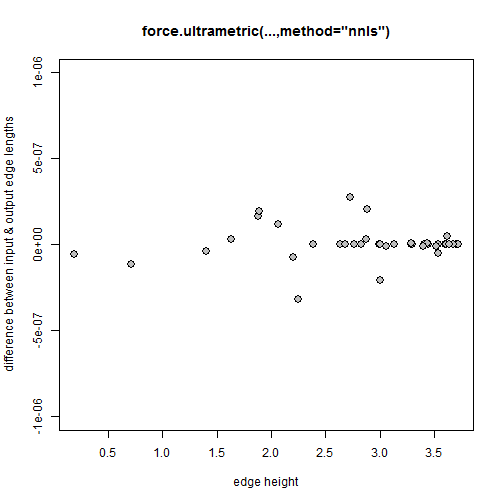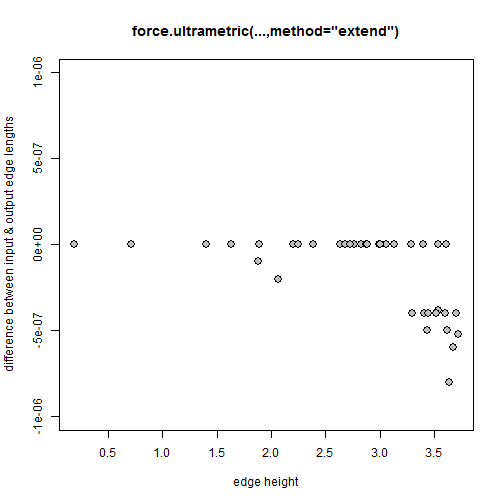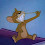## Tuesday, March 28, 2017

### `force.ultrametric` method for ultrametric phylogenies that fail `ape::is.ultrametric` due to numerical precision

Sometimes in R a phylogenetic tree will fail the check `is.ultrametric` even though we know our tree is ultrametric. Why?

Generally speaking, this is because a tree in R is actually never* precisely ultrametric due to limitations in numerical precision inherent to all computer machinery. (*The exception is when our tree has integer branch lengths, in which case it could be exactly ultrametric.)

Consequently, `is.ultrametric` has an argument `tol = .Machine\$double.eps0.5` which sets the tolerance level - basically how much deviation from precise ultrametricity (is this a word?) should be permitted.

Unfortunately, sometimes trees that should be ultrametric that have been written to file & then read into R will fail this test with `tol` set at its default value. In addition, because the default value for `tol` is a function of the maximum numerical precision of the machine, this value can theoretically vary between computers & R versions!

Here is a quick example of how a tree that seems to be ultrametric can fail a check of `is.ultrametric`:

``````library(phytools)
set.seed(1)
tree<-pbtree(n=26,tip.label=LETTERS)
text<-write.tree(tree,digits=7)
text
``````
``````##  "(A:3.759964,(B:3.382373,((((C:0.327977,D:0.327977):0.8764073,(((E:0.1249564,F:0.1249564):0.6915993,(G:0.707227,H:0.707227):0.1093287):0.2328474,(I:0.9354844,(J:0.3184016,K:0.3184016):0.6170828):0.1139187):0.1549812):0.3387382,(L:0.6247575,M:0.6247575):0.918365):1.172667,(((N:0.6491293,(O:0.08390608,P:0.08390608):0.5652232):0.4727952,((Q:0.172787,R:0.172787):0.1103943,S:0.2831814):0.8387431):0.8701228,((T:0.3105662,U:0.3105662):1.438824,(((V:0.4557458,W:0.4557458):0.02627092,X:0.4820167):0.7931008,(Y:0.2386761,Z:0.2386761):1.036441):0.4742723):0.2426576):0.7237421):0.6665833):0.3775909);"
``````
``````tree<-read.tree(text=text)
plotTree(tree)
````````````is.ultrametric(tree)
``````
``````##  FALSE
``````

I don't know about you - but I have certainly seen a lot of 'ultrametric' trees written to file with 7 digit precision!

Here is a very simple function that will force our “nearly ultrametric” tree to be precisely ultrametric. It basically wraps two different methods. One is the function `nnls.tree` in the package phangorn by Klaus Schliep in my lab. For a given tree, this function can find the set of edge lengths with implied distances with minimum sum-of-squared differences to the true distances - in this case the patristic distances on our phylogeny.

The second method is very simple. It just computes the total amount of edge length that would have to be added to all the tips of tree to render the tree ultrametric. This seems nice, but it will concentrate the fudge we are applying to our tree edges to external edges only. I believe that this method is also implemented in a popular package called BioGeoBEARS by Nick Matzke.

Here is that function:

``````force.ultrametric<-function(tree,method=c("nnls","extend")){
method<-method
if(method=="nnls") tree<-nnls.tree(cophenetic(tree),tree,
rooted=TRUE,trace=0)
else if(method=="extend"){
h<-diag(vcv(tree))
d<-max(h)-h
ii<-sapply(1:Ntip(tree),function(x,y) which(y==x),
y=tree\$edge[,2])
tree\$edge.length[ii]<-tree\$edge.length[ii]+d
} else
cat("method not recognized: returning input tree\n\n")
tree
}
``````

Now we can try it:

``````library(phangorn)
ult.nnls<-force.ultrametric(tree) ## default method
is.ultrametric(ult.nnls)
``````
``````##  TRUE
``````
``````ult.extend<-force.ultrametric(tree,method="extend")
is.ultrametric(ult.extend)
``````
``````##  TRUE
``````

Now let's compare the edge lengths of each of these two trees, as a function of edge height, to the edge lengths of our input tree:

``````ult.nnls<-reorder(ult.nnls)
h.nnls<-rowMeans(nodeHeights(ult.nnls))
plot(h.nnls,tree\$edge.length-ult.nnls\$edge.length,pch=21,
ylim=c(-1e-6,1e-6),bg="grey",cex=1.5,xlab="edge height",
ylab="difference between input & output edge lengths",
main="force.ultrametric(...,method=\"nnls\")")
````````````sum((tree\$edge.length-ult.nnls\$edge.length)^2)
``````
``````##  3.673085e-13
``````
``````h.extend<-rowMeans(nodeHeights(ult.extend))
sum((tree\$edge.length-ult.extend\$edge.length)^2)
``````
``````##  5.2996e-12
``````
``````plot(h.extend,tree\$edge.length-ult.extend\$edge.length,pch=21,
ylim=c(-1e-6,1e-6),bg="grey",cex=1.5,xlab="edge height",
ylab="difference between input & output edge lengths",
main="force.ultrametric(...,method=\"extend\")")
``````I'd say that the `"nnls"` method looks better.

Note that neither of these is a formal statistical method for estimating an ultrametric tree from a tree in which branch lengths are proportional to (for instance) molecular sequence evolution - there are a number of different approaches for doing this. Rather, this method is designed merely to 're-adjust' the edge lengths of a tree that has lost numerical precision from being written to file & thus fails R checks such as `is.ultrametric`.

1.Hi Liam,

Great post and nice function! Here're a few comments.

is.ultrametric() has the option 'option' to choose the method used to test ultrametricity: the default is the scaled range. The other possibility is the variance (option = 2) which used to be the default before ape 4.0.

To avoid issues with the number of printed digits in write.tree/nexus, one can use readRDS instead: it makes smaller files that are faster to read/write, and no digits are lost. Of course, you won't be able to open such files with other software than R.

A third way to make a tree ultrametric: branching.times() does not test whether the tree is ultrametric, so the values output by this function from an "almost ultrametric" tree can then be input to compute.brtime().

Cheers,

Emmanuel

1.Thanks Emmanuel.

2.For context, I should add that Emmanuel discovered this post when I shared it in a bammtools issue discussion regarding recent changes to is.ultrametric in ape, which may be relevant to this discussion:

https://github.com/macroevolution/bammtools/issues/45

1.Thank you Dave. I hope you are well.

3.We offer you all world famous replica watches, with same appearance, same quality and same material…you cannot tell which one is a replica-rolex just with your eyes at all!

4.if any of these options works? what should I do?

Note: due to the very large amount of spam, all comments are now automatically submitted for moderation.